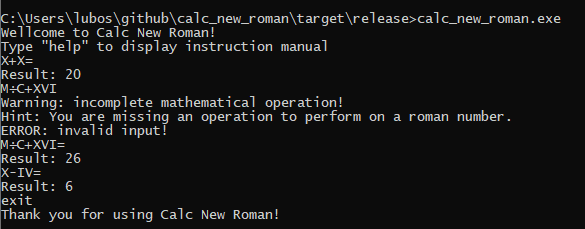# Calc New Roman

Current version: unreleased

Calc New Roman is an open-source text-based calculator for working with Roman numbers.commands:

• help – display this manual
• exit – exit application

Application usage: you can input roman numbers with the mathematical operations you want to perform. State that your mathematical operation is finished by putting “=” in it’s last place. Note: support for correct ordering of mathematical operations is not yet implemented. Operations are executed in the order in which you put them in. There are 4 basic principles for writing Roman numerals as listed below:

The letters I, X, C can be repeated thrice in succession. Additionally, L, V, D cannot be repeated or the number is considered to be invalid. If a lower value digit is written to the left of a higher value digit, it is subtracted. If a lower value digit is written to the right of a higher value digit, it is added. Only I, X, and C can be used as subtractive numerals.